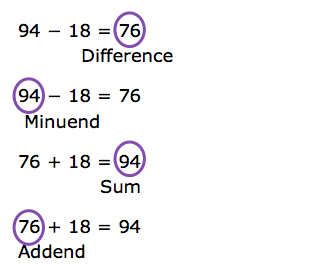Subtrahend

My Definition

Key Characteristics

The following are true of a subtrahend:

• A subtrahend is the number being subtracted from another number or quantity.

• Subtrahends may be whole numbers, fractions, decimals, integers, real numbers, and imaginary numbers.

• Subtrahends may be polynomials.

Example

Use the link below to further study examples of a subtrahend.

Subtrahend Activity

Non-example